## Example Questions

← Previous 1 3 4 5 6 7 8 9 10

### Example Question #1 : How To Find An Angle In A Right Triangle

Acute angles x and y are inside a right triangle. If x is four less than one third of 21, what is y?

87

18

3

90

7

87

Explanation:

We know that the sum of all the angles must be 180 and we already know one angle is 90, leaving the sum of x and y to be 90.

Solve for x to find y.

One third of 21 is 7. Four less than  7 is 3. So if angle x is 3 then that leaves 87 for angle y.

### Example Question #2 : Right Triangles

If a right triangle has one leg with a length of 4 and a hypotenuse with a length of 8, what is the measure of the angle between the hypotenuse and its other leg?

30

45

65

90

60

30

Explanation:

The first thing to notice is that this is a 30o:60o:90o triangle. If you draw a diagram, it is easier to see that the angle that is asked for corresponds to the side with a length of 4. This will be the smallest angle. The correct answer is 30.

### Example Question #3 : Right Triangles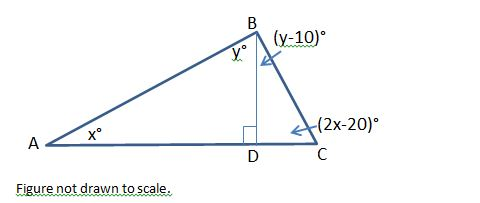In the figure above, what is the positive difference, in degrees, between the measures of angle ACB and angle CBD?

50

30

20

40

10

10

Explanation:

In the figure above, angle ADB is a right angle. Because side AC is a straight line, angle CDB must also be a right angle.

Let’s examine triangle ADB. The sum of the measures of the three angles must be 180 degrees, and we know that angle ADB must be 90 degrees, since it is a right angle. We can now set up the following equation.

x + y + 90 = 180

Subtract 90 from both sides.

x + y = 90

Next, we will look at triangle CDB. We know that angle CDB is also 90 degrees, so we will write the following equation:

y – 10 + 2x – 20 + 90 = 180

y + 2x + 60 = 180

Subtract 60 from both sides.

y + 2x = 120

We have a system of equations consisting of x + y = 90 and y + 2x = 120. We can solve this system by solving one equation in terms of x and then substituting this value into the second equation. Let’s solve for y in the equation x + y = 90.

x + y = 90

Subtract x from both sides.

y = 90 – x

Next, we can substitute 90 – x into the equation y + 2x = 120.

(90 – x) + 2x = 120

90 + x = 120

x = 120 – 90 = 30

x = 30

Since y = 90 – x, y = 90 – 30 = 60.

The question ultimately asks us to find the positive difference between the measures of ACB and CBD. The measure of ACB = 2x – 20 = 2(30) – 20 = 40 degrees. The measure of CBD = y – 10 = 60 – 10 = 50 degrees. The positive difference between 50 degrees and 40 degrees is 10.

### Example Question #4 : Right Triangles

Which of the following sets of line-segment lengths can form a triangle?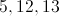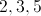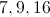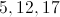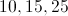Explanation:

In any given triangle, the sum of any two sides is greater than the third.  The incorrect answers have the sum of two sides equal to the third.

### Example Question #2 : How To Find An Angle In A Right Triangle

In right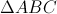,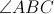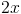and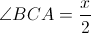.

What is the value of?

24

30

48

32

36

36

Explanation:

There are 180 degrees in every triangle. Since this triangle is a right triangle, one of the angles measures 90 degrees.

Therefore,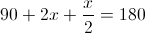.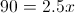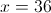### Example Question #6 : Right Triangles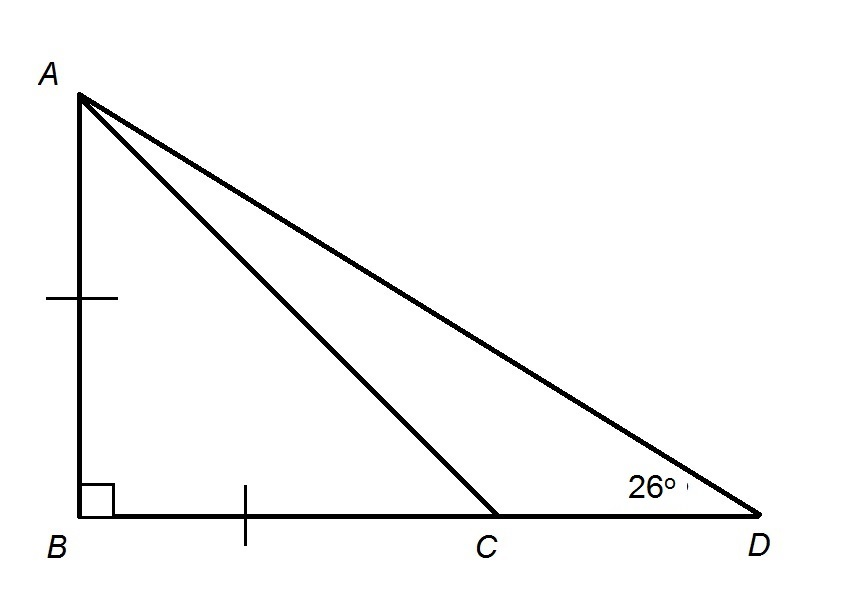Figure is not drawn to scale

Refer to the provided figure. Evaluate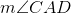.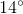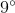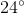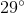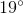Explanation: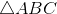is an isosceles right triangle with right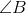, so, by the 45-45-90 Triangle Theorem,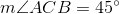. This angle is an exterior angle to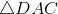,  so its measure is equal to the sum of those of its two remote interior angles,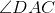and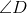. That is,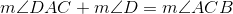Settingand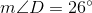, solve for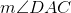: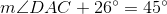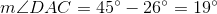### Example Question #7 : Right TrianglesFigure not drawn to scale.

In the figure above, rays PA and PB are tangent to circle O at points A and B, respectively. If the diameter of circle O is 16 units and the length of line segment PO is 17 units, what is the area, in square units, of the quadrilateral PAOB?

60

120

136

240

68

120

Explanation:

Because PA and PB are tangent to circle O, angles PAO and PBO must be right angles; therefore, triangles PAO and PBO are both right triangles.

Since AO and OB are both radii of circle O, they are congruent. Furthermore, because PA and PB are external tangents originating from the same point, they must also be congruent.

So, in triangles PAO and PBO, we have two sides that are congruent, and we have a congruent angle (all right angles are congruent) between them. Therefore, by the Side-Angle-Side (SAS) Theorem of congruency, triangles PAO and PBO are congruent.

Notice that quadrilateral PAOB can be broken up into triangles PAO and PBO. Since those triangles are congruent, each must comprise one half of the area of quadrilateral PAOB. As a result, if we find the area of one of the triangles, we can double it in order to find the area of the quadrilateral.

Let's determine the area of triangle PAO. We have already established that it is a right triangle. We are told that PO, which is the hypotenuse of the triangle, is equal to 17. We are also told that the diameter of circle O is 16, which means that every radius of the circle is 8, because a radius is half the size of a diameter. Since segment AO is a radius, its length must be 8.

So, triangle PAO is a right triangle with a hypotenuse of 17 and a leg of 8. We can use the Pythagorean Theorem in order to find the other leg. According to the Pythagorean Theorem, if a and b are the lengths of the legs of a right triangle, and c is the length of the hypotenuse, then:

a2 + b2 = c2

Let us let b represent the length of PA.

82 + b2 = 172

64 + b2 = 289

Subtract 64 from both sides.

b2 = 225

Take the square root of both sides.

b = 15

This means that the length of PA is 15.

Now let's apply the formula for the area of a right triangle. Because the legs of a right triangle are perpendicular, one can be considered the base, and the other can be considered the height of the triangle.

area of triangle PAO = (1/2)bh

= (1/2)(8)(15) = 60

Ultimately, we must find the area of quadrilateral PAOB; however, we previously determined that triangles PAO and PBO each comprise half of the quadrilateral. Thus, if we double the area of PAO, we would get the area of quadrilateral PAOB.

Area of PAOB = 2(area of PAO)

= 2(60) = 120 square units

### Example Question #8 : Right Triangles

If the hypotenuse of a triangle is 5 meters, which of the following is the closest value to the area of the triangle?

5

45

54

26

12

12

Explanation:

The answer is 12. In this circumstance, the area of the triangle cannot be smaller than its hypotenuse length, and cannot be bigger than its hypotenuse squared (that would be the area of a square).

### Example Question #9 : Right Triangles

Triangle ABC is drawn between the points A(4, 3), B(4, 8), and C(7, 3). What is the area of ABC?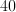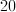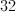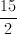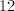Explanation:

Drawing a quick sketch of this triangle will reveal that it is a right triangle. The lines AB and AC form the height and base of this triangle interchangeably, depending on how you look at it.

Either way the formula for the area of the triangle is the distance from A to B multiplied by the distance from A to C, divided by 2.

This is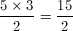### Example Question #10 : Right Triangles

A right triangle has a total perimeter of 12, and the length of its hypotenuse is 5. What is the area of this triangle?

6

10

15

12

3

6

Explanation:

The area of a triangle is denoted by the equation 1/2 b x h.

b stands for the length of the base, and h stands for the height.

Here we are told that the perimeter (total length of all three sides) is 12, and the hypotenuse (the side that is neither the height nor the base) is 5 units long.

So, 12-5 = 7 for the total perimeter of the base and height.

7 does not divide cleanly by two, but it does break down into 3 and 4,

and 1/2 (3x4) yields 6.

Another way to solve this would be if you recall your rules for right triangles, one of the very basic ones is the 3,4,5 triangle, which is exactly what we have here

← Previous 1 3 4 5 6 7 8 9 10